# cytofWorkflow之基本质量控制（三）

``````require(cytofWorkflow)
p <- plotExprs(sce, color_by = "condition")
p\$facet\$params\$ncol <- 6
p
``````

### 再次回顾一下SingleCellExperiment对象构建的代码

``````require(cytofWorkflow)

if(!file.exists(file = 'input_for_cytofWorkflow.Rdata')){
url <- "http://imlspenticton.uzh.ch/robinson_lab/cytofWorkflow"
table(md[,3:4])
# 样本的表型信息

## 真正的表达矩阵
library(HDCytoData)
fs <- Bodenmiller_BCR_XL_flowSet()
fs
# 如果网络不好，也可以自行下载
# 然后：loaded into R as a flowSet using read.flowSet() from the flowCore package

# 这个cytof的panel的抗体信息表格：
panel <- "PBMC8_panel_v3.xlsx"

# spot check that all panel columns are in the flowSet object
all(panel\$fcs_colname %in% colnames(fs))

# 有了样本的表型信息，panel的抗体信息，以及表达量矩阵，就可以构建对象：
# specify levels for conditions & sample IDs to assure desired ordering
md\$condition <- factor(md\$condition, levels = c("Ref", "BCRXL"))
md\$sample_id <- factor(md\$sample_id,
levels = md\$sample_id[order(md\$condition)])
save(fs, panel, md,file = 'input_for_cytofWorkflow.Rdata')

}

# expression values, 第一个样品是2838个细胞，39列（其中6列并不是抗体）
dim(exprs(fs[]))
exprs(fs[])[1:6, 1:5]
colnames(exprs(fs[]))
# construct SingleCellExperiment
panel
md
library(CATALYST)
sce <- prepData(fs, panel, md, features = panel\$fcs_colname)

pro='basic_qc'

p <- plotExprs(sce, color_by = "condition")
p\$facet\$params\$ncol <- 4
p
ggsave2(filename = paste0(pro,'_all_markers_density.pdf'))
``````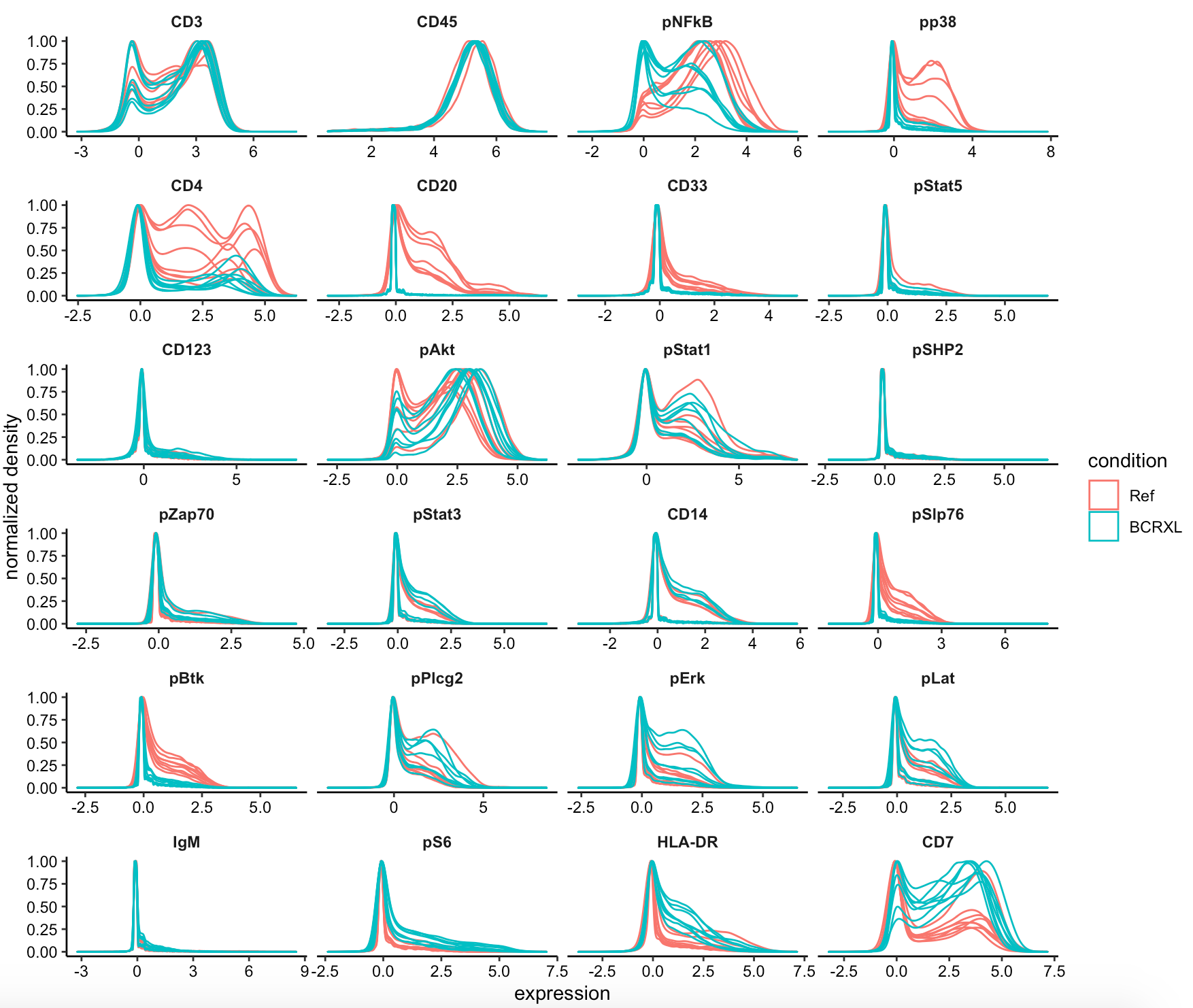### 然后看看2个分组的各自8个样品的细胞数量

``````n_cells(sce)
plotCounts(sce, group_by = "sample_id", color_by = "condition")
ggsave2(filename = paste0(pro,'_plotCounts.pdf'))
``````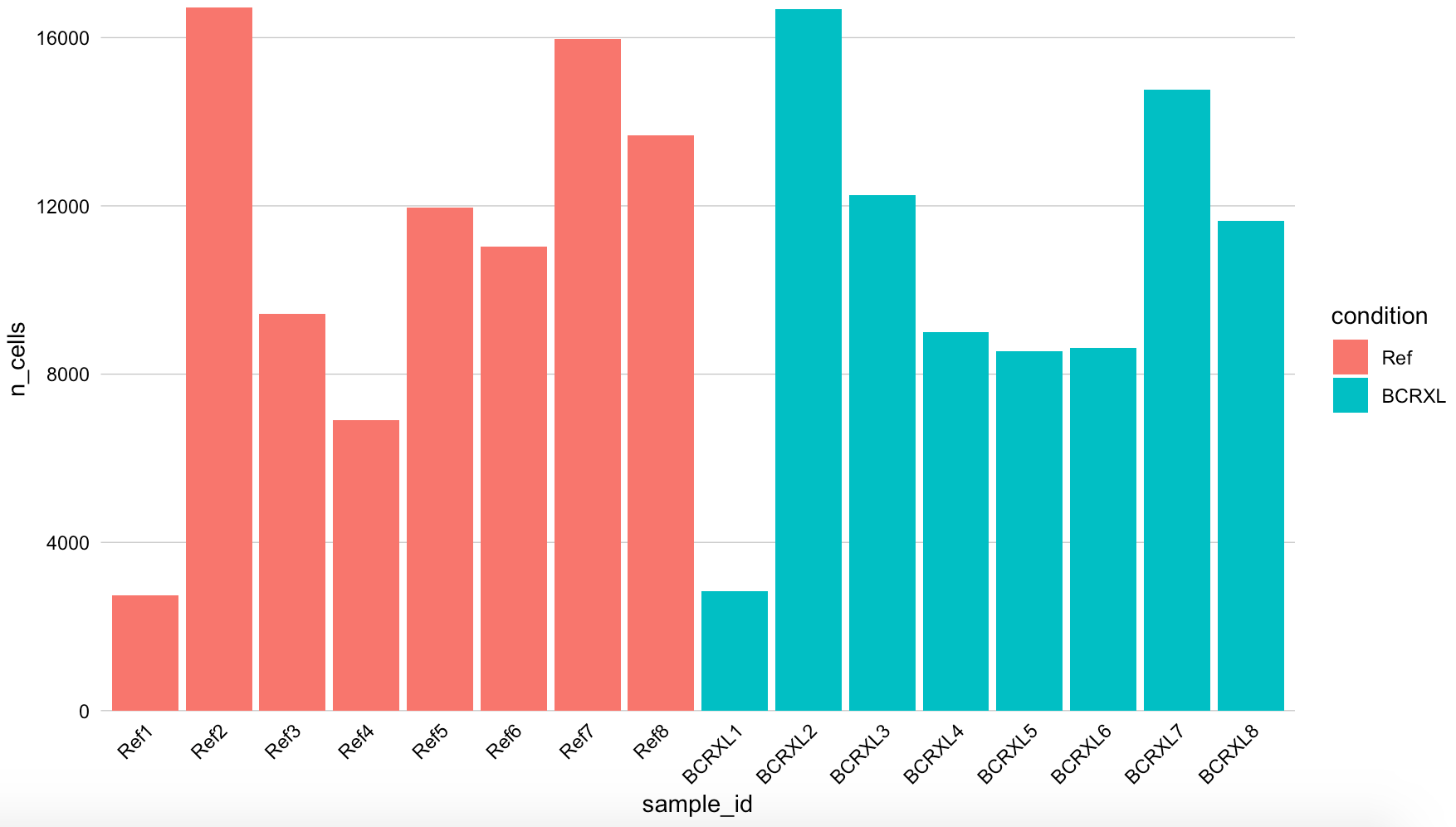``````df=data.frame(n_cells=unlist(lapply(1:length(fs), function(i){
dim(exprs(fs[[i]]))
})),
condition=md\$condition,sample_id=md\$sample_id)
library(ggplot2)
ggplot2::ggplot(df,aes(x=sample_id,y=n_cells,fill=condition))+ geom_bar(stat = "identity")
``````

### 然后看看样品之间的距离关系

``````# pbMDS: Pseudobulk-level MDS plot.
# A multi-dimensional scaling (MDS) plot on aggregated measurement values may be rendered with pbMDS.
pbMDS(sce, color_by = "condition", label_by = "sample_id")

ggsave2(filename = paste0(pro,'_pbMDS.pdf'))
``````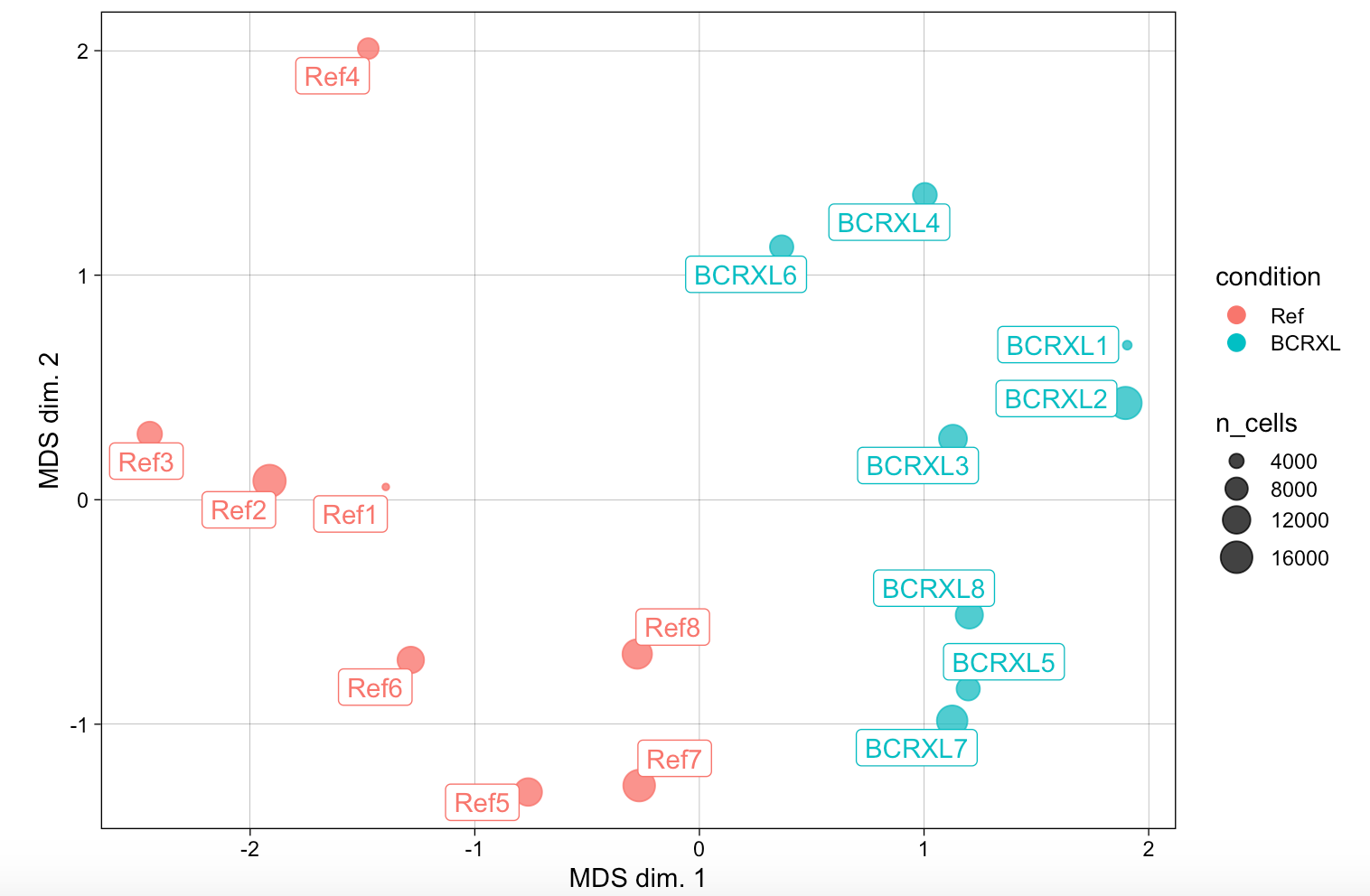### 表达量热图看差异

``````# 10 lineage markers and 14 functional markers across all cells measured for each sample in the PBMC dataset
pdf( paste0(pro,'_plotExprHeatmap.pdf'))
plotExprHeatmap(sce, scale = "last",
hm_pal = rev(hcl.colors(10, "YlGnBu")))
dev.off()
``````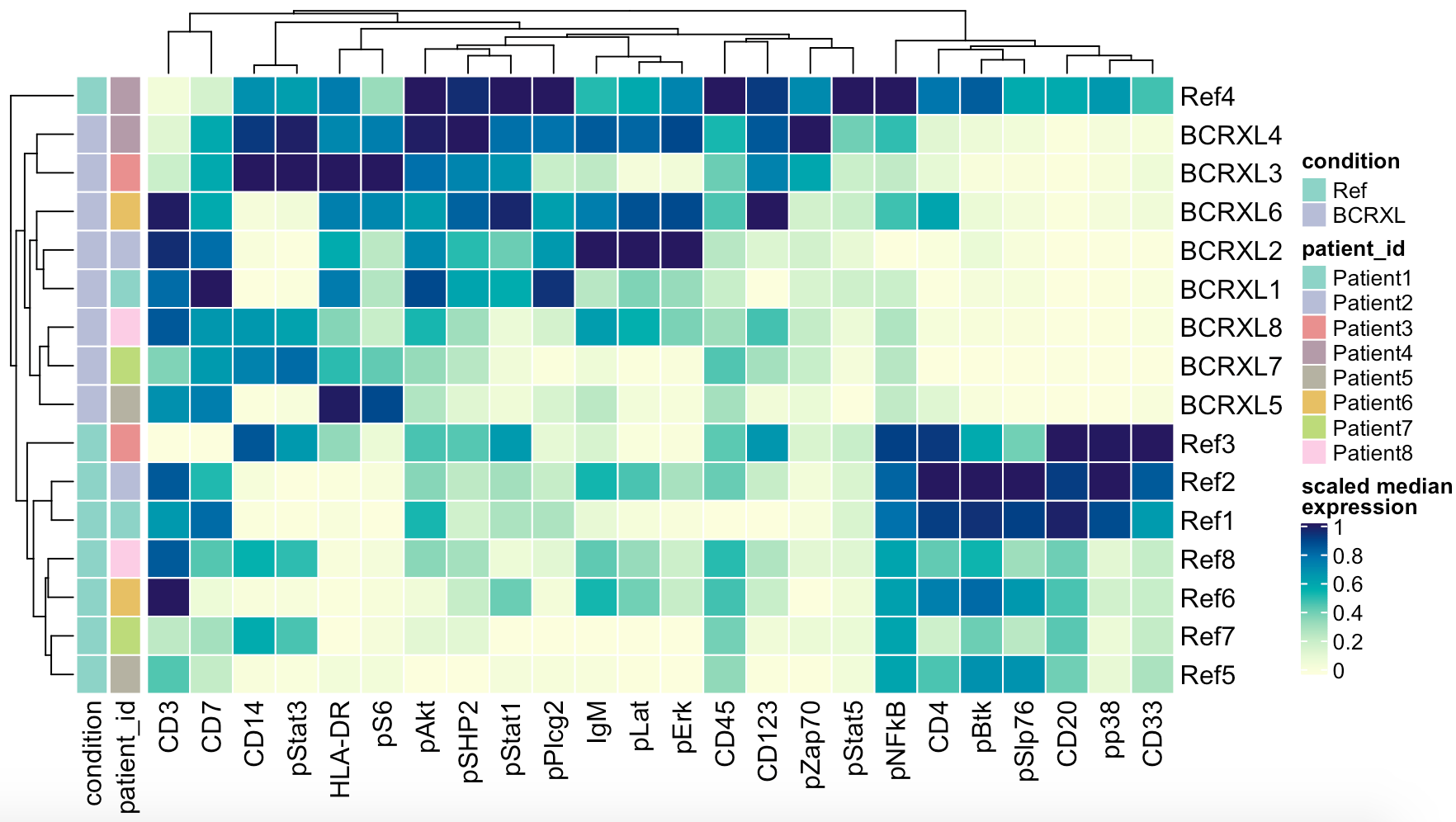### 另外一种展现表达量差异的条形图

``````# PCA-based non-redundancy score (NRS)
plotNRS(sce, features = "type", color_by = "condition")
ggsave2(filename = paste0(pro,'_plotNRS.pdf'))
``````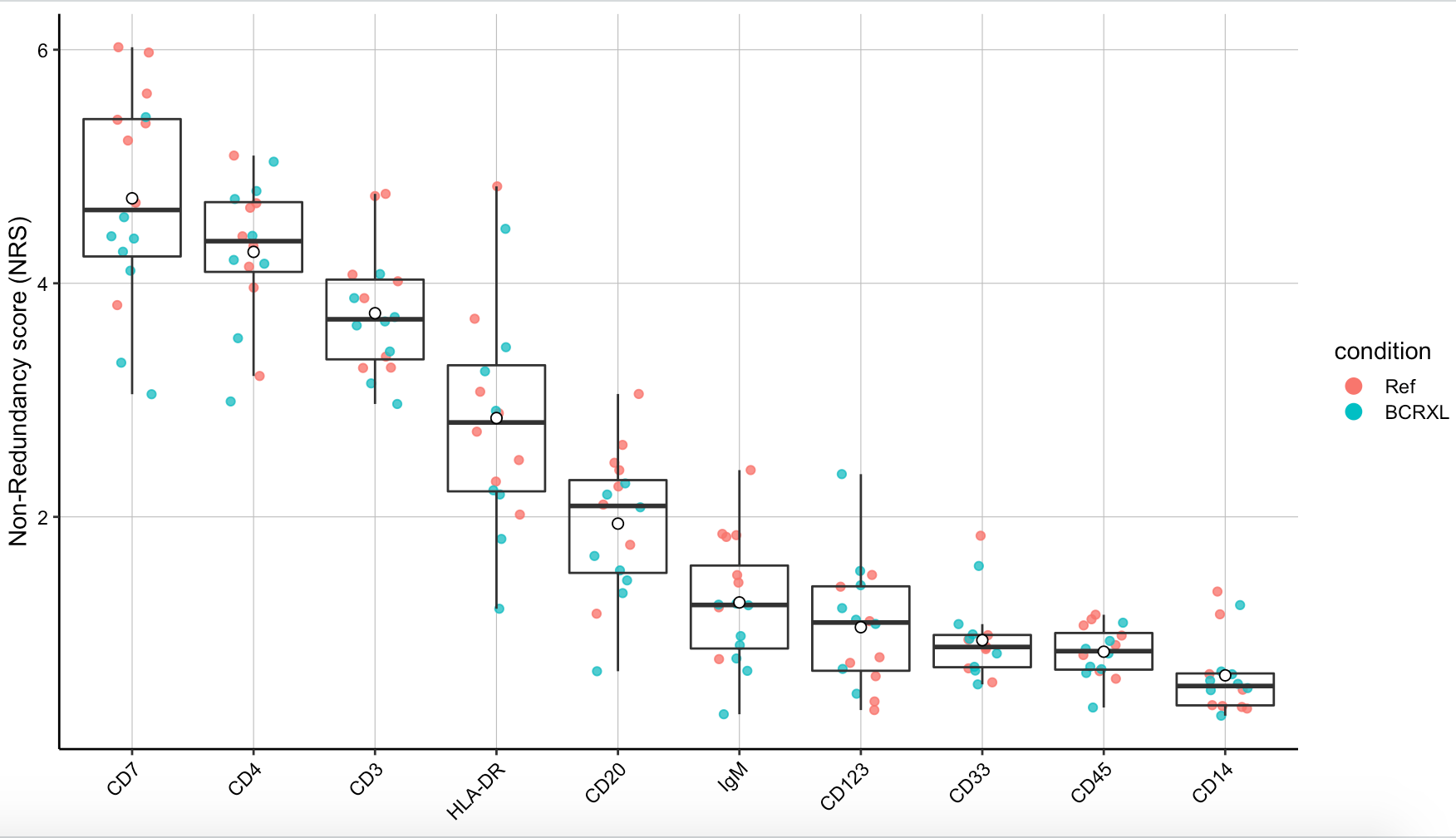PCA-based non-redundancy score 比较难理解，反正绝大部分情况下我是忽略这张图的，虽然说cytofWorkflow教程里面展现了它。